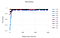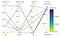# Tune your Channel Attribution Model

How do the hyperparameters affect your attribution model and how do you choose which ones to tune?

In our previous blog, we introduce how we use attention mechanism for marketing attribution to relate different positions of a sequence of touch points. However, the performance of the model can depend on the selection of hyperparameters.

In this blog, we explain how we use Azure Machine Learning to select the best model in order to have an accurate representation of marketing performance.

Let’s start!

# What is hyperparameter tuning?

In machine learning, hyperparameter optimization or tuning is the problem of choosing a set of optimal hyperparameters for a learning algorithm. Hyperparameters are adjustable parameters that let you control the model training process. For example, with neural networks, we can choose different number of hidden layers, we can change the learning rate, the number of epochs or the batch size. The model performance depends heavily on hyperparameters. Therefore, they need to be tuned so that the model can optimally solve the machine learning problem.

Hyperparameter tuning is accomplished by training multiple models, using the same algorithm and training data but with different hyperparameter values. Then each training run is evaluated and the best-performing model is selected.

# What is Azure Machine Learning?

Azure Machine Learning is a cloud-environment used to train, deploy, manage and track machine learning models. There are three ways to develop machine learning models:

1. The Automated ML component which allows to automatically train a model based on a target metric by simply ingesting a dataset and the ML task to be executed.
2. The Designer which allows users to visually build ML workflows by dragging and dropping pre-made ML tasks into a canvas and connecting them.
3. The SDKs (Python and R) which allow to build and run ML workflows from Azure ML’s built-in notebook functionality.

Azure Machine Learning revolves around the concept of creating end-to-end machine learning pipelines and experiments.

• Pipelines: Pipelines are independently executable workflows of complete ML tasks. Subtasks are encapsulated as a series of steps within this pipeline, covering whatever content the user wants to execute. Pipelines can be created by using the SDK for Python or the Designer functionality.
• Experiments: Whenever a pipeline is run, the outputs will be submitted to an experiment in the Azure ML workspace. These experiments bundle runs together in one interface, which makes it easier to compare the results of the ML model development.

# Getting Started

First of all, we need to set up our workspace. Once the new workspace is configured, we need to set up a datastore to access the data . Each workspace has a default datastore. In this blog, we won’t explain how to set up the workspace. However, more information can be found here.

Once our workspace and datastore are configured. We proceed to define important parameters required to run our experiments:

# Defining a search space

The set of hyperparameter values tried during hyperparameter tuning is known as the search space. Hyperparameters can be:

• Discrete — these hyperparameters require discrete values, that is, we must select the value from a particular set of possibilities.
• Continuous — we can use any value along a scale or interval.

# Sampling the hyperparameter space

The values that we will use during the different runs depend on the type of sampling:

• Grid sampling can only be employed when all hyperparameters are discrete, and is used to try every possible combination of parameters in the search space.
• Random sampling, as its name suggests, is used to randomly select values. They can be a discrete or continuous.
• Bayesian sampling select parameter combinations that will result in improved performance from the previous selection.

# Early termination policy

With a sufficiently large hyperparameter search space, it could take many iterations. To help prevent wasting time, we set up an early termination policy. This parameter automatically end poorly performing runs to improve computational efficiency.

# Specify metric

A metric goal is used to determine whether a higher value for a metric is better or worse. This metric can be accuracy, f-score, precision, recall, among others. We specify the metric we can to optimise. In our experiments we used accuracy. Each run will be evaluated for the metric goal and the early termination policy uses this metric to identify low-performance runs.

There are other parameters that can be configured. However, we just explained the most relevant to our work.

Now we can configure our hyperparameter tuning experiment. We make use of ScriptRunConfig, which is the training script that will run with the sampled hyperparameters. Here we specify the configuration details of the training job. This includes the training, environment to use, and the compute target to run on. Here is where we specify the parameter sampling method to use over the hyperparameter space. In our experiment, we use RandomParameterSampling.

The parameters we will control are: batch size, learning rate, epochs, training size, padding length.

Then, the job is submitted via HyperDriveConfig.

Now we able to select and use the best model for our attribution model. We can visualise our hyperparameter tuning runs in Azure Machine Learning studio or we can use a notebook widget.

With this graph, we can track the performance of each child run. Each line represents a child run, and each point measures the accuracy at each iteration.Accuracy performance for each child run

With this graph, we can see which combination of hyperparameters has the best performance. It plots the correlation between accuracy and individual hyperparameters values.Correlation between accuracy and individual hyperparameters values

# Conclusion

In this blog, we explained how we use Azure Machine Learning for hyperparameter optimisation. We are able to improve the performance of our model and by selecting the best model we are confident in using the best results to inform and drive our marketing strategy.

The QueryClick R&D team’s blog of exciting projects they’ve worked on recently